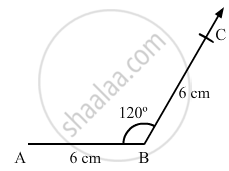Advertisement Remove all ads

# Construct a Quadrilateral Abcd in Which Ab = Bc = 6 Cm, Ad = Dc = 4.5 Cm and ∠B = 120°. - Mathematics

Answer in Brief

Construct a quadrilateral ABCD in which AB = BC = 6 cm, AD = DC = 4.5 cm and ∠B = 120°.

Advertisement Remove all ads

#### Solution

Steps of construction:

Step I: Draw AB = 6 cm .

Step II: Construct ABC = 120°.

Step III : With B as the centre and radius 6 cm, cut off BC = 6 cm .

Now, we can see that AC is about 10 . 3 cm which is greater than AD + CD = 4 . 5 + 4 . 5 = 9 cm .

We know that sum of the lengths of two sides of triangle is always greater than the third side but here, the sum of AD and CD is less than AC .

So, construction of the given quadrilateral is not possible .Is there an error in this question or solution?
Advertisement Remove all ads

#### APPEARS IN

RD Sharma Class 8 Maths
Chapter 18 Practical Geometry (Constructions)
Exercise 18.3 | Q 6 | Page 8
Advertisement Remove all ads

#### Video TutorialsVIEW ALL 

Advertisement Remove all ads
Share
Notifications

View all notifications

Forgot password?
Course